# Civil Engineering - UPSC Civil Service Exam Questions

26.

A thin cylindrical shell of internal diameter D is subject to an internal pressure 'p'. If σy is the yield stress of the material of the cylinder, then based on the maximum shear theory, the thickness of the cylinder will be (in kg/cm2) is

 A.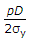B.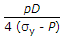C.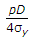D.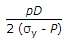Explanation:

No answer description available for this question. Let us discuss.

27.

A shaft of diameter 'd' is subjected to bending moment 'M' and twisting moment 'T' The developed principal stress will be

 A.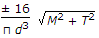B.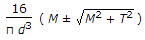C.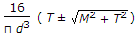D.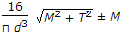Explanation:

No answer description available for this question. Let us discuss.

28.

There are ten instrument staions occupied in succession during a traverse survey. An observer makes equal error in each station, the magnitude of which is δ θ in each instance at all the stations. What is the probable error of the final bearing at the end of the traverse ?

 A. ± 10 δ θ B. ± 100(δ θ)2 C. ± 10 (δ θ) D. ± l0 δ θ

Explanation:

No answer description available for this question. Let us discuss.

29.

At a certain station, the mean of the average temperature is 25°C and mean of the maximum daily temperature is 40°C. What is the airport reference temperature (ART) ?

 A. 46.6°C B. 45°C C. 35°C D. 30°C

Explanation:

No answer description available for this question. Let us discuss.

30.

The area between the two isohytes 45 cm and 55 cm is 100 km2, and that between 55 cm and 65 cm is 150 km2. What is the average depth of annual precipitation over the basin of 250 km2 ?

 A. 50 cm B. 52 cm C. 56 cm D. 60 cm